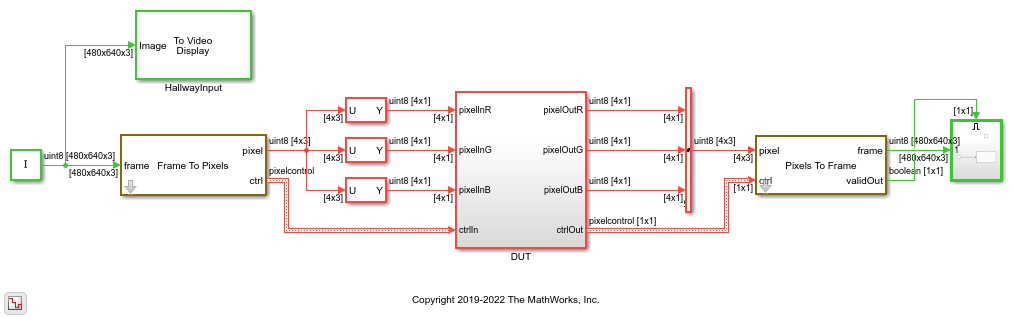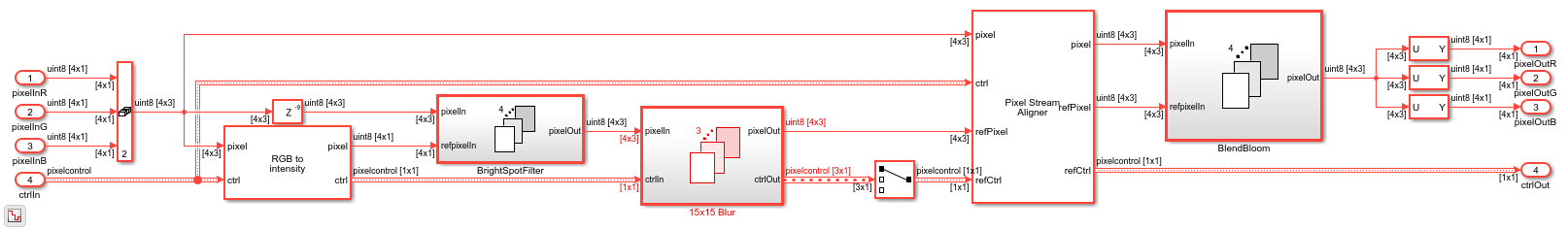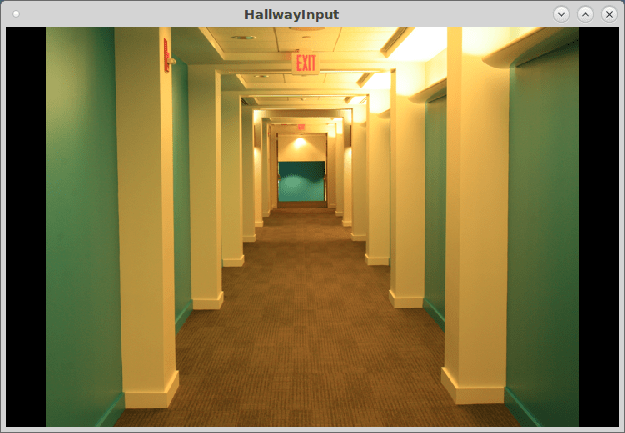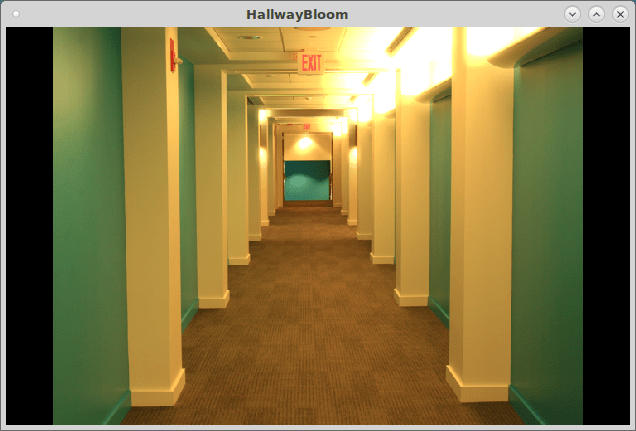Main Content

# MultiPixel-MultiComponent Video Streaming

This example shows how to work with a multipixel-multicomponent pixel stream. Multipixel-multicomponent streaming enables real-time processing of high-resolution or high-frame-rate color video streams.

To demonstrate working with such a video stream, this example implements the well-known bloom effect image post-processing technique. The bloom effect introduces or enhances the glow of light sources in an image.

### Top Level I/0

Each pixel of a high-resolution or high-frame-rate pixel stream is modeled as a NumPixel-by-NumComponent matrix. Matrix data types are supported for HDL code generation within a design, but not for the ports of the top-level subsystem. In this case, the input pixel stream is split into three 4-by-1 vectors at the input of the DUT, and then recombined at the output into a 4-by-3 matrix for the Pixels To Frame block.### Bloom Effect

The example model follows these three steps to add a bloom effect to the input image.

1. The MultiPixelColorSpaceConverter and BrightSpotFilter subsystems find bright spots in the intensity image by checking pixel values against a threshold.

2. The 15x15 Blur subsystem spreads out the bright spots by applying a Gaussian filter.

3. The BlendBloom subsystem adds the Gaussian-enhanced bright spots back to the original image.

The model uses For Each subsystems for repeated operations in parallel, which results in a cleaner model and reduces the number of HDL files that are generated.### Matrix Flattening

Vision HDL Toolbox™ neighborhood-processing blocks can operate on vector inputs, but do not support matrix inputs. The line buffer used inside the blocks returns a NumPixels-by-KernelHeight matrix. Using multicomponent inputs would result in a NumPixels-by-KernelHeight-by-NumComponents output matrix, however, 3-D matrices are not supported for HDL code generation. For Each subsystems support HDL code generation with scalar and vector inputs but not with matrix inputs. To work around this issue, the model flattens the NumPixels-by-NumComponents matrix into a NumPixels*NumComponents-by-1 vector, and then uses the partition settings on For Each subsystems to repeat the operation across each slice of the vector.

The model encodes the pixel vector in two different ways. The first encoding represents the pixels as four RGB values concatenated together into a 12-by-1 vector, as shown in this diagram. The model sets the Partition width parameter of the MultiPixelColorSpaceConverter subsystem to `3`. The subsystem implements four RGB-to-Intensity operations in parallel.The second pixel encoding concatenates four R, G, or B pixels together, as shown in this diagram. The 15x15 Blur subsystem accepts a 12-by-1 input vector. The model sets the Partition width parameter of the 15x15 Blur subsystem to `4`, so it operates on three 4-by-1 multipixel vectors. The subsystem implements three Image Filter blocks in parallel. Each filter operates on a 4-by-15 window and returns a 4-by-1 vector.### Simulation Results

Simulating the model displays these input and output images. The bloom effect makes the lighted areas of the scene look brighter and shows a halo effect.### Implementation Results

This table shows the synthesis results of HDL code generated from the DUT subsystem and synthesized for a Xilinx™ Zynq™ ZC706 board. Because none of the resources exceed 25% of their respective category, the design has a relatively small footprint.

```T = 4x2 table Resource Usage _________ _____ DSP48 84 Flip Flop 62724 LUT 36710 BRAM 132 ```

## SupportGet trial now